Alert: You are not allowed to copy content or view source
Register for Maths, Science, English, GK Olympiad Exam Click here | Check Olympiad Exam Dates here | Buy Practice Papers for IMO, IOM, HEO, IOEL etc here | Login here to participate in all India free mock test on every Saturday

# Introduction to QBasic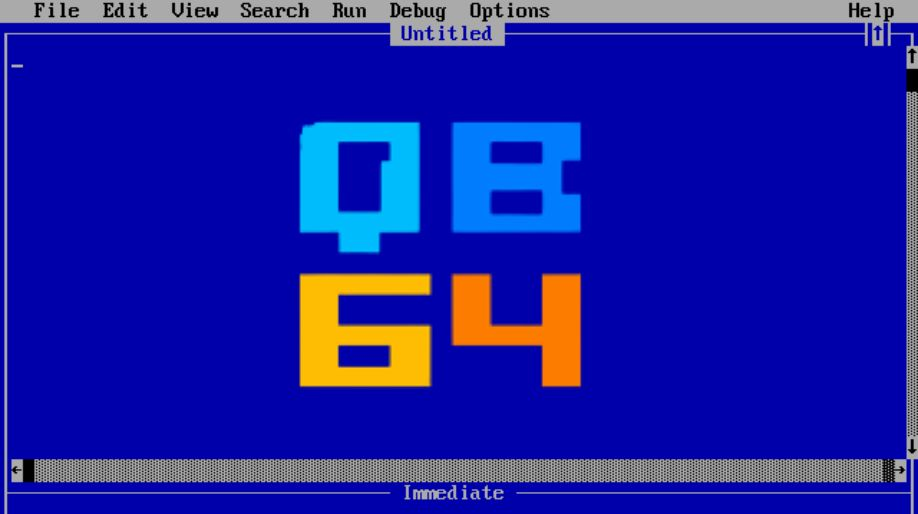Let's learn about a programming language called QBasic today.

• With respect to class 6 Introduction to QBasic, QBasic refers to Quick Beginners All purpose Symbolic Instruction Code.
• It is a simple programming language developed by Microsoft to type, edit, debug and execute BASIC programs.
• It is used in the MS-DOS operating system.
• QBasic uses English like words and Mathematical symbols to write programs. It is very easy and simple to understand.
• The extension of a QBasic program file is “.bas “.

## With respect to class 6 Introduction to QBasic, what are the features of QBasic?

With respect to class 6 computer lessons, QBasic is a high level programming language. The features of QBasic are:

1. QBasic is very easy and simple to understand
2. QBasic automatically checks syntax.
3. QBasic capitalizes the reserved words.
4. QBasic keeps the same variable name used in a program to identical form.
5. QBasic allows you to break lengthy programs into modules.

## With respect to class 6 Introduction to QBasic, Elements Of QBasic

A QBasic program consists of various elements. They are: a character set, constants, variables, statements, operators and expressions.

CHARACTER SET

With respect to class 6 computer lessons, the QBasic Character Set consists of:

• Alphabets from A-Z and a-z.
• Numbers from 0 to 9 and
• Special characters such as +, -, *, <, >, =, ', ", /, ^, #, \$, %, etc.

CONSTANTS

With respect to class 6 computer lessons, a constant is the fixed value which is used in the program. They are two types:

1. Numeric Constants: Numeric constant is a numeric value which is used in a program. For example -104, 102.50, 710456, etc.
2. String Constants: String constant is a sequence of characters which is used in a program. In other words, it is the collection of numbers or texts or a combination of both text and numbers. For example - "Hello", “Computer “, etc.

VARIABLE

With respect to class 6 computer lessons, a variable is a location in the memory, which has been assigned a name, and it used to store data temporarily. It holds the value until another value is assigned to it.

There are two types of variables:

1. Numeric variables: It can hold only numeric value. For example A, B, A1, ABC, C_4, etc.
2. String variables: It is represented by an alphabet followed by the dollar (\$) sign. It contains letters, digits, underscore but the last character is always a \$. For example B3\$, OP1\$, etc.

OPERATOR

With respect to class 6 Introduction to QBasic, operators are symbols that indicate the type of operation QBasic has to perform on the data or on the values of variables.

There are three types of operators in QBasic. They are:

1. Mathematical Operators: The table contains the types of Mathematical operators available in QBasic.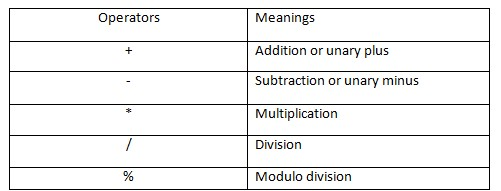1. Relational Operators: The table contains the types of relational operators available in QBasic.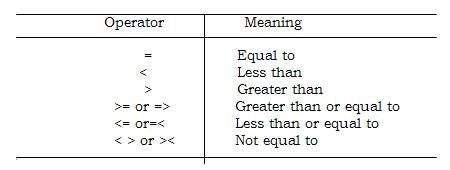• Logical Operators: The table contains the types of logical operators available in QBasic.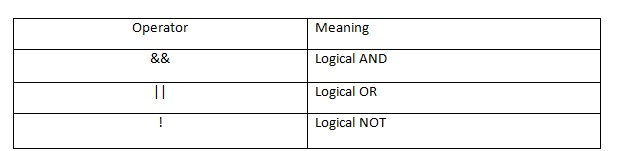EXPRESSIONS

With respect to class 6 computer lessons, an expression is the combination of three elements of QBasic. They are- operators, constants and variables. They are evaluated to get a result. The result of the expression can be stored in a variable. For example - (A + B) > C

## QBasic Statement

With respect to class 6 computer lessons, a QBasic statement is a command or set of instructions to perform certain tasks. The followings are QBasic statement along with their uses –

1. CLS:

CLS command is used to clear the screen.

1. PRINT:

It is used to print the values of variables.

Example-

CLS

X=10

PRINT "The value of X is "; X

END

The output of the given program will be

The value of X is 10

1. END:

It ends the execution of the program.

Example-

CLS

U= 14

PRINT U

END

1. INPUT:

It allows us to enter a value from the keyboard and assign the entered value in a variable.

Example-

CLS

INPUT "Enter the first number";x

INPUT "Enter a second number";y

S=x+y

PRINT "Sum of two numbers is "; S

END

1. REM

REM statement is used to write remarks or comments in the program. Remarks are added in a program only for the understanding purpose, it is not executed in the program.

Example-

CLS

REM program calculates the area of a square

INPUT "Enter a length"; L

A=L^2

PRINT "Area of a square is "; A

END

## Examples with respect to class 6 Introduction to QBasic:

1. ____ uses English like words and Mathematical symbols to write programs.

Ans: QBasic.

1. QBasic allows you to break lengthy programs into?

Ans: Modules.

1. What is the fixed value used in the program?

Ans: Constants.

1. What holds a value until another value is assigned to it?

Ans: Variable.

1. ____ is a command or set of instructions to perform certain tasks.

Ans: Statement.

## Starting With QBasic

With respect to class 6 computer lessons, QBasic can be started using the following steps:

1. Click the Start button.
2. Go to All Programs and then Accessories. And open command prompt window.
3. In command prompt window, select the drive and directory where the QBasic application is installed.
4. Type QBasic and press Enter.
5. Press Esc key to get QBasic Editor Screen.

QBasic Editor Screen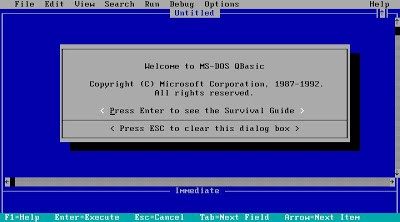QBasic editor is the window where QBasic programs are written. The QBasic Editor Screen has three parts. They are-

1. Editor window – Programs are typed in the editor window.
2. Immediate window- It is used while correcting errors in a program.
3. Output window- The output window is used to display the output of the program.

Running a Program

To execute a program, Press Shift + F5 key or press Alt+ R.

Saving a Program

With respect to class 6 Introduction to QBasic, a program in QBasic is saved by-

1. Click the File menu and then click Save As. The Save As dialog box appears.
2. Enter the file name. And click OK.

Exiting QBasic

To exit from the QBasic editor window –

Press ALT + F key. Press X or select Exit command under the File menu and press Enter key.

## Recap with respect to class 6 Introduction to QBasic:

• QBasic is a simple programming language developed by Microsoft to type, edit, debug and execute BASIC programs.
• A QBasic program consists of various elements. They are a character set, constants, variables, statements, operators and expressions.
• A QBasic statement is a command or set of instructions to perform a certain task. Certain statements used in QBasic- CLS, INPUT, PRINT, END and REM.
• QBasic editor is the window where QBasic programs are written. The QBasic Editor Screen has three parts. They are- Editor Window, Immediate Window and Output Window.

## Quiz for Introduction to QBasic

 Q.1 What is the default Program name? a) Untitled b) Document1 c) Basic1 d) File1
 Q.2 It is the actual workspace where you type a program: a) Immediate Mode Area b) Scroll bar c) Program Space d) Program Name
 Q.3 Which bar helps us to navigate through the document? a) Immediate Mode Area b) Scroll bar c) Program Area d) Program Name
 Q.4 It is the mode in which QBASIC statement is executed as soon as the Enter key is pressed: a) Immediate Mode Area b) Scroll bar c) Program Area d) Program Name
 Q.5 This command helps to clear the contents of the screen: a) CLEAR b) PLS c) CLS d) CLR
 Q.6 Displays the output on the screen: a) CLEAR b) PLS c) CLS d) PRINT
 Q.7 To execute or to run a QBASIC program click on _____________ menu under RUN option. a) EXECUTE b) START c) RUN d) CONTINUE
 Q.8 What is the shortcut to run a program? a) Shift + F5 b) Shift + F2 c) Shift + F7 d) Shift + F9
 Q.9 Click on Open option under: a) View b) Edit c) File d) Search
 Q.10 To open a new Document we need to click on New in which menu? a) View b) Edit c) File d) Open